# 深度学习

Machine Learning - Android Ng## 3.1. 神经网络概论

• 尝试模仿（mimic）人脑

• 用处

• speech -> images -> text(NLP) -> …
• 每个神经元接受一些输入，做一些计算，然后将输出送给下一个神经元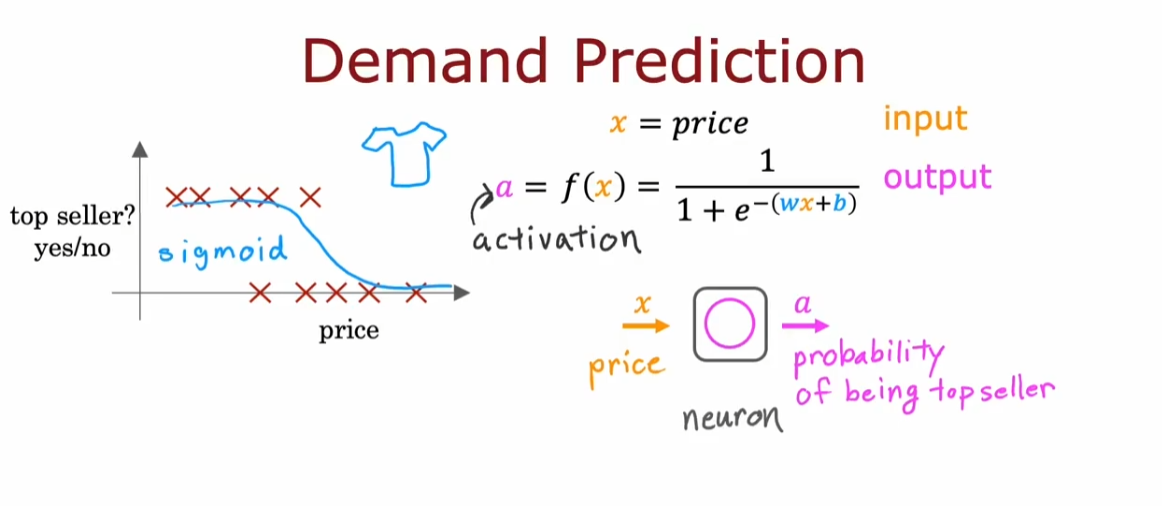• 可以将神经元分为不同层（layer），每层接受相似的输入，输出不同的结果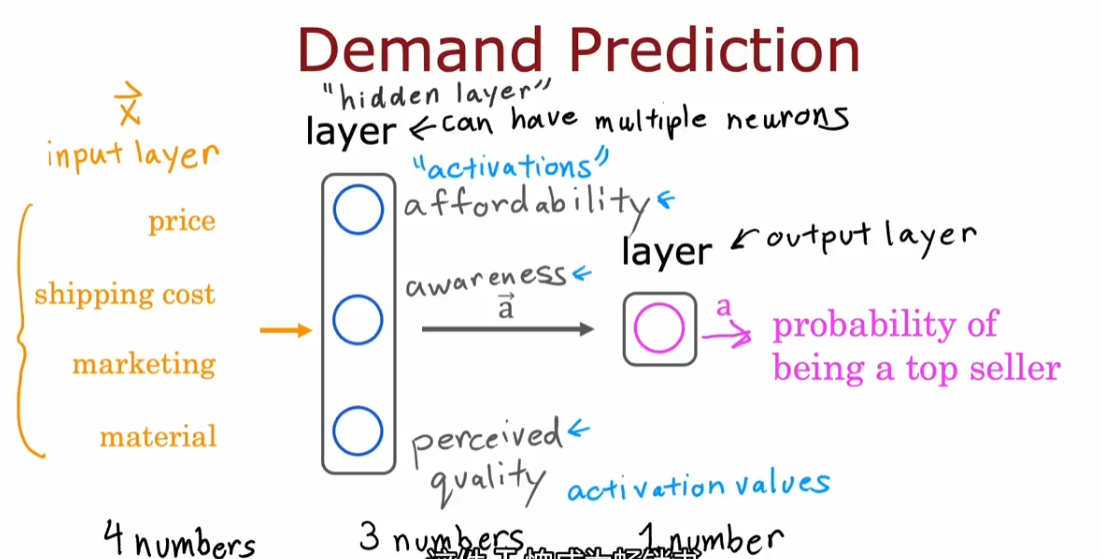• 神经网络不需要自己进行特征工程，中间的隐藏层即通过输入初始特征来输出更好的特征

• 需要自己决定的是神经网络的架构：即有多少层，每层有多少神经元

### 3.1.1. 一些 notation

• 方括号上标代表第 x 层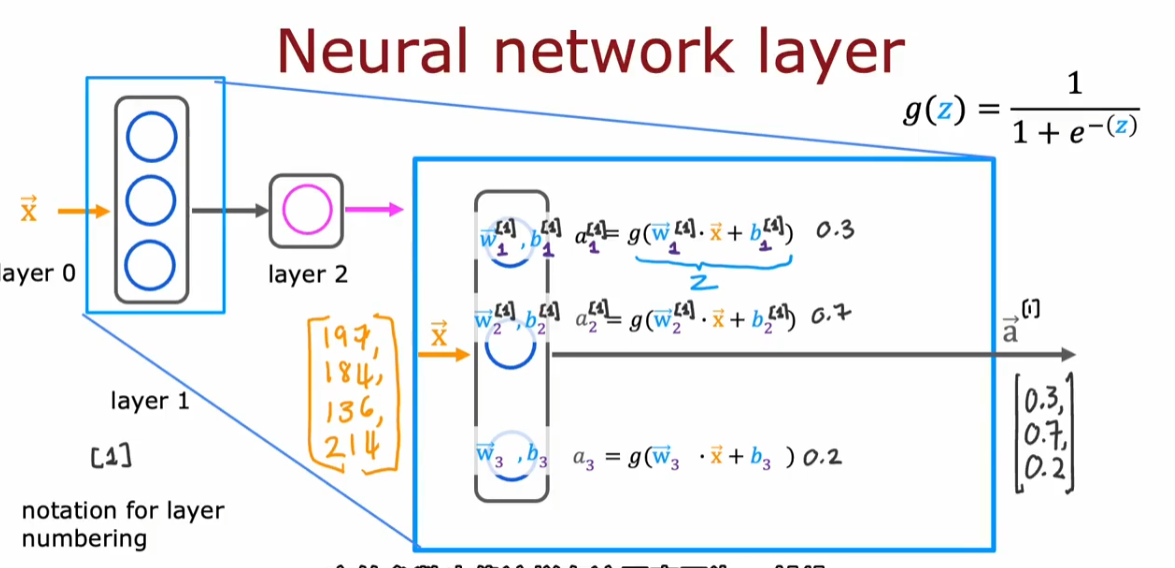• 每层接受的输入向量上标是上一层的
• $a^{}$一般表示输入向量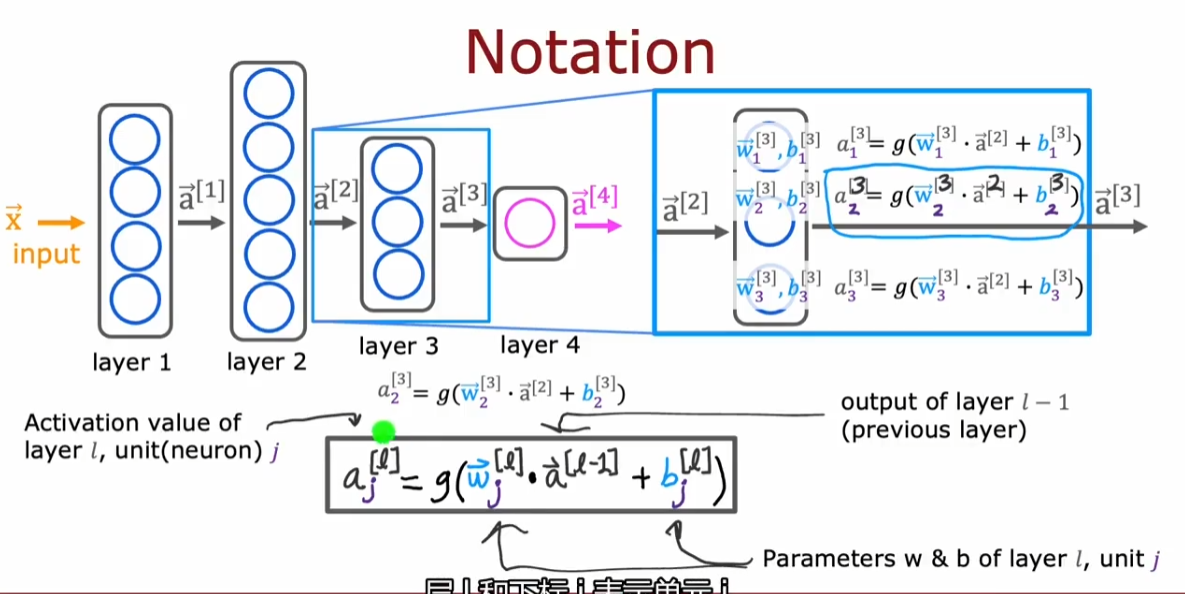• 前向传播
• 从神经网络层自左向右传播

## 3.2. TensorFlow 介绍

### 3.2.2. Tf 的数据格式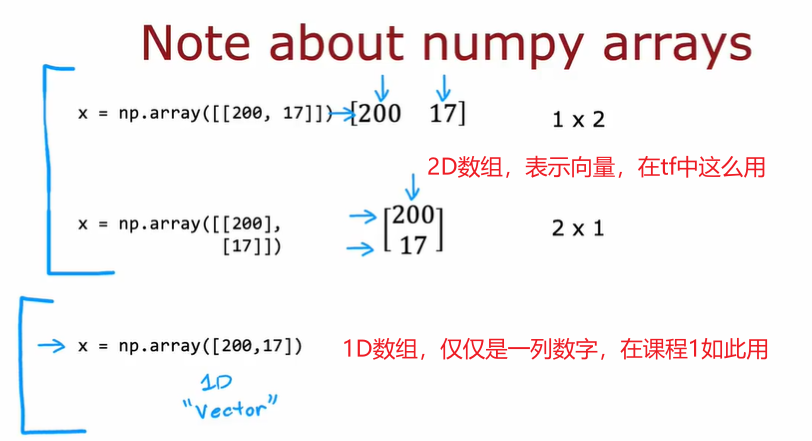### 3.2.3. Tensor（张量）

• 可以近似理解为一种矩阵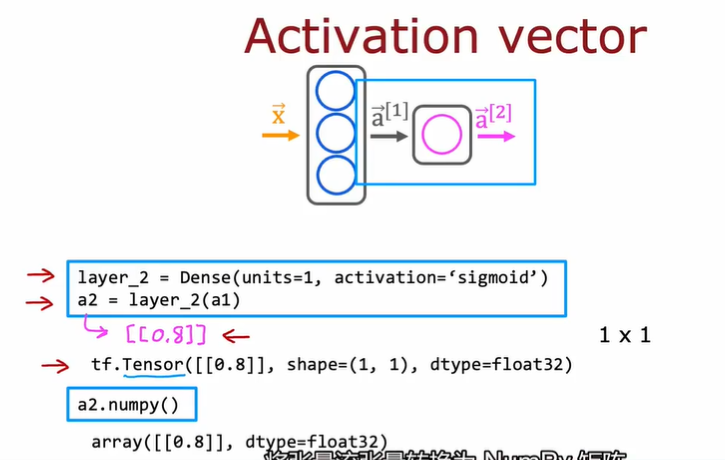## 3.3. Python 向前传播的原理

• forward prop
• 手写全连接层（full connected）

## 3.4. AI 和神经网络的关系

### 3.4.1. ANI 和 AGI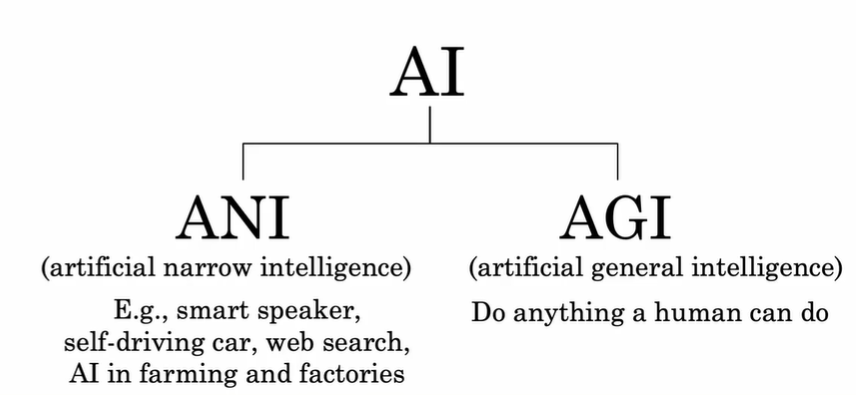### 3.4.2. The “one learning algorithm” hypothesis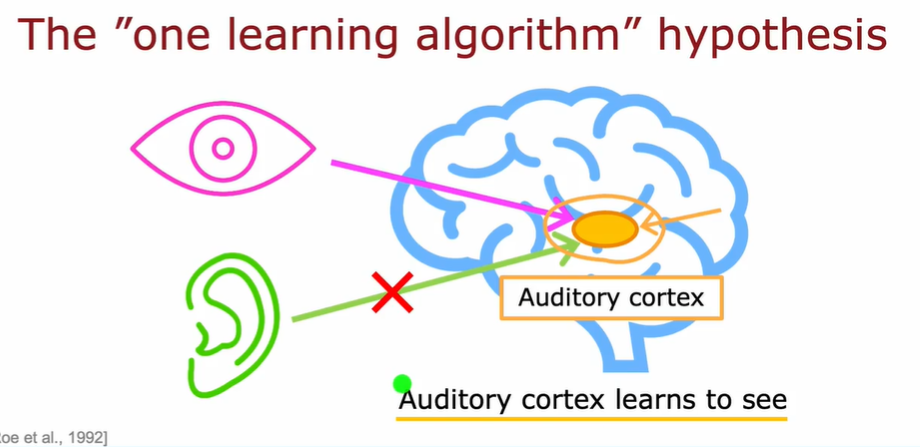## 3.5. 算法中的向量化实现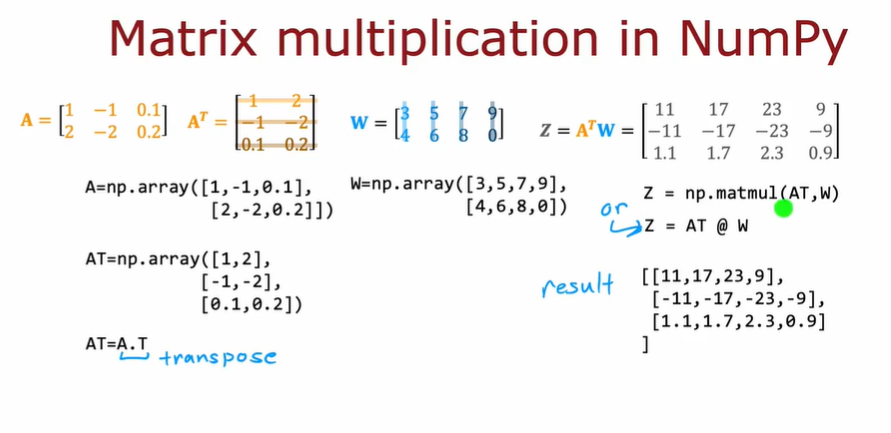• 使用矩阵和向量运算的好处是：可以利用硬件进行并行计算，显著提高运算速度

• 在 TensorFlow 的约定中：一个样本的数据通常在矩阵的行中

## 3.6. TensorFlow 实现

• tf 编译模型的关键是定义损失函数
• 第一步：定义模型
• 第二步：使用损失函数编译模型
• 第三步：训练模型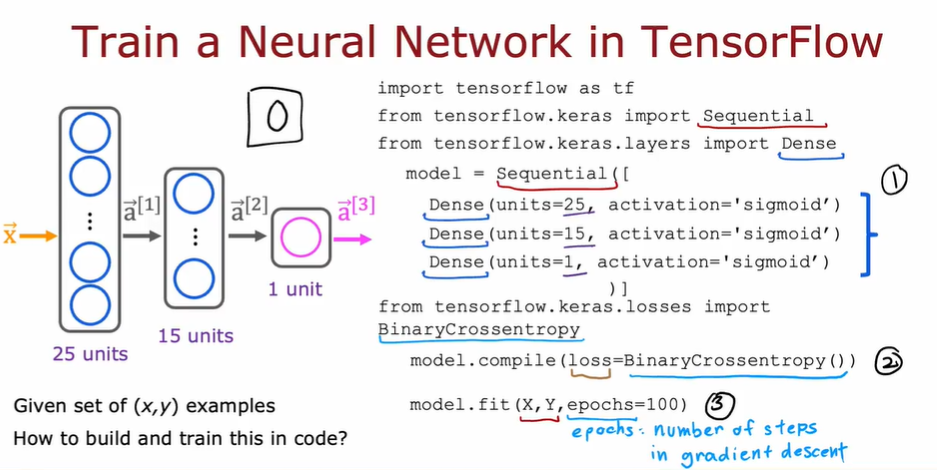### 3.6.1. 前述基本步骤在 tf 中的对应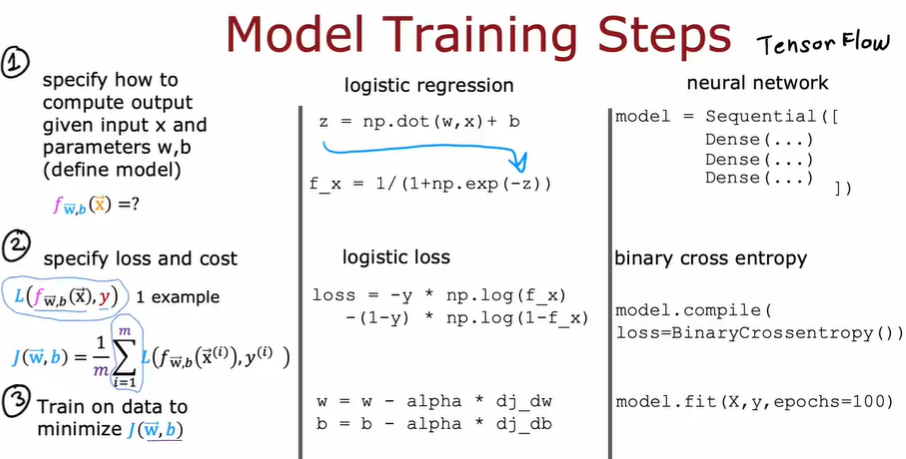• tf 的 model.fi 通过反向传播实现了梯度下降

## 3.7. 激活函数详解

• 一些激活函数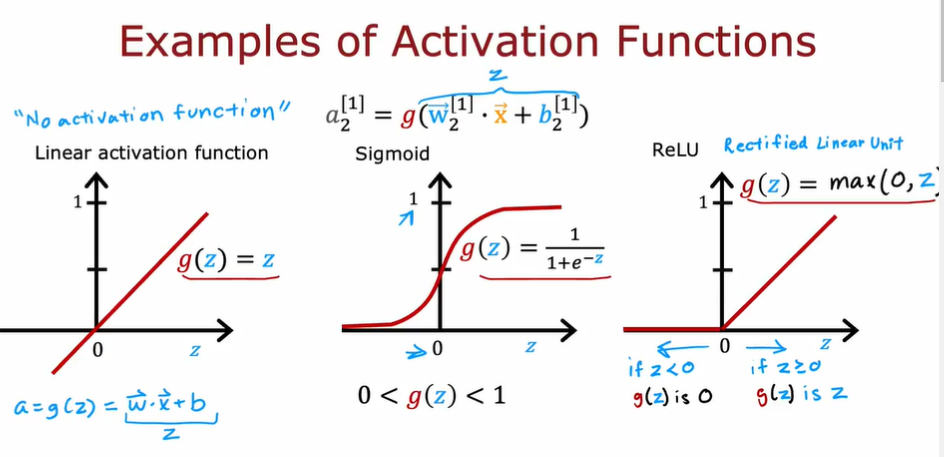### 3.7.1. ReLU

• Rectified Linear Unit 修正线性单元
• 计算速度比 sigmoid 更快
• 只有左端是趋于 flat 的，因此在梯度下降时比 sigmoid 更有优势，学习速度更快
• 一般作为隐藏层的默认激活函数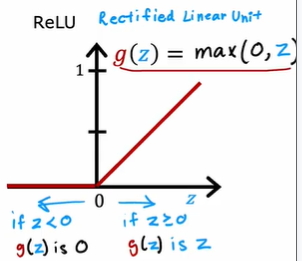### 3.7.2. softmax

• 逻辑回归的泛化
• 针对多分类环境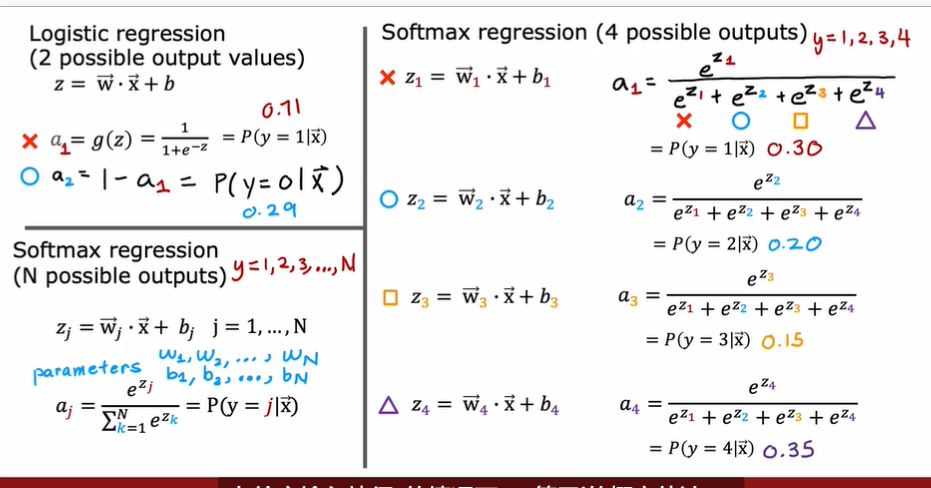#### 3.7.2.1. softmax 函数的损失函数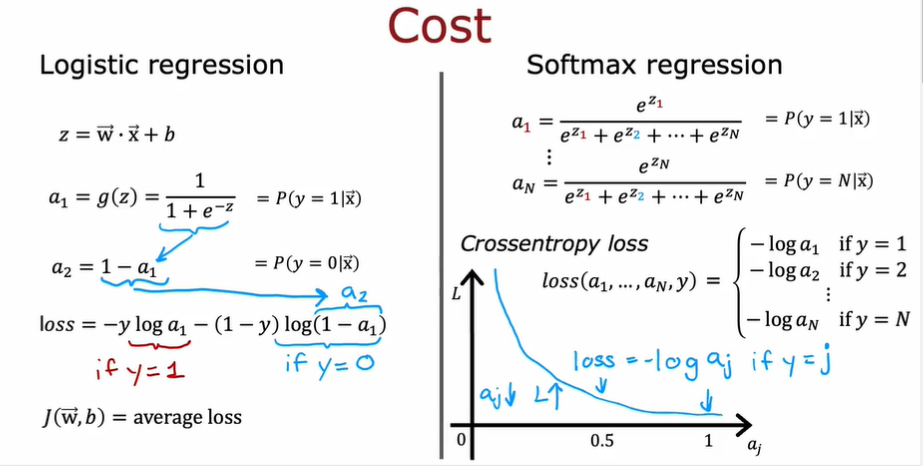• 可以和 sigmoid 类比，$a_j$越接近 1，损失越小，这会刺激$a_j$不断接近 1

### 3.7.3. 如何选择激活函数

• sigmoid：二元分类问题，因为神经网络在学习 y=1 的概率
• linear：回归
• ReLU：回归，且结果是非负值

### 3.7.4. 为什么我们需要激活函数

• 简单来说，如果我们不使用激活函数，那么所有神经网络将变成如同线性回归般的简单计算。多层神经网络不能提供提升计算复杂特征的能力，也不能学习任何比线性函数更复杂的东西，违背了我们创造神经网络的初衷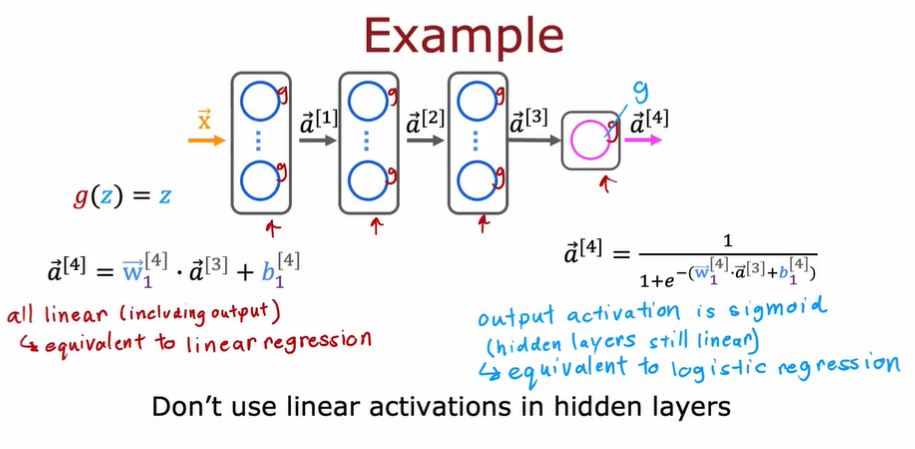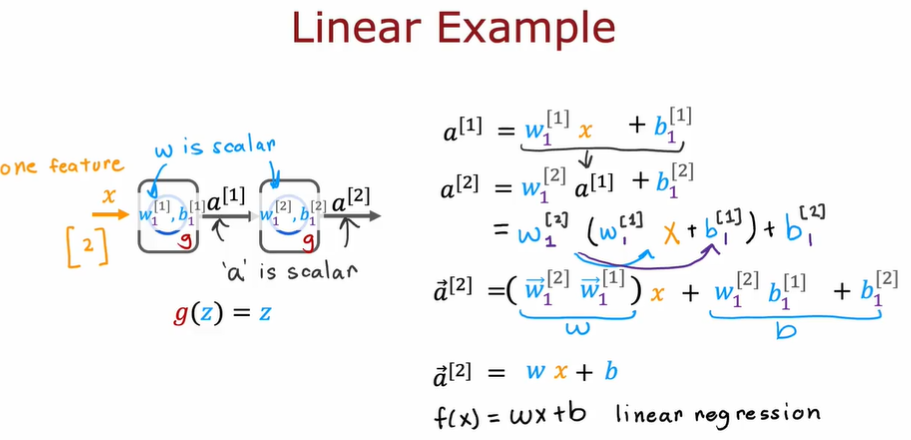## 3.8. 多分类

• y 是离散的，且可能的取值大于 2 种
• 使用 softmax 激活函数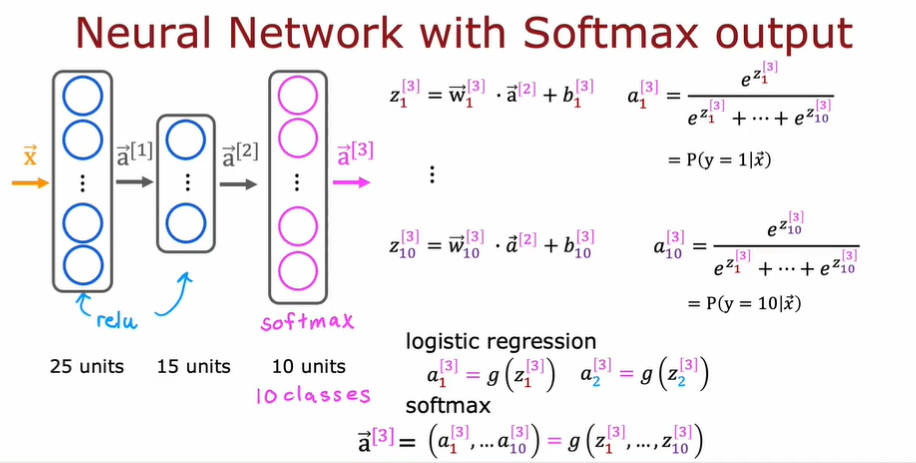• softmax 函数在这里和其他激活函数的区别是，softmax 函数一次性算出$a_1, a_2, …, a_i$的所有值，并计算出它们的概率。而其他激活函数仅仅只是一次计算出一个。

### 3.8.1. 使用 tf 实现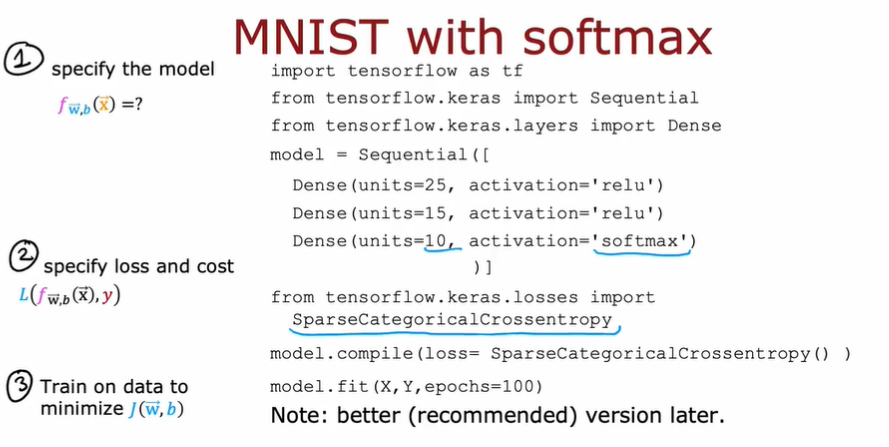• 一种 tf 实现的版本，但是实际上有更好的版本。因此

#### 3.8.1.1. 优化计算精度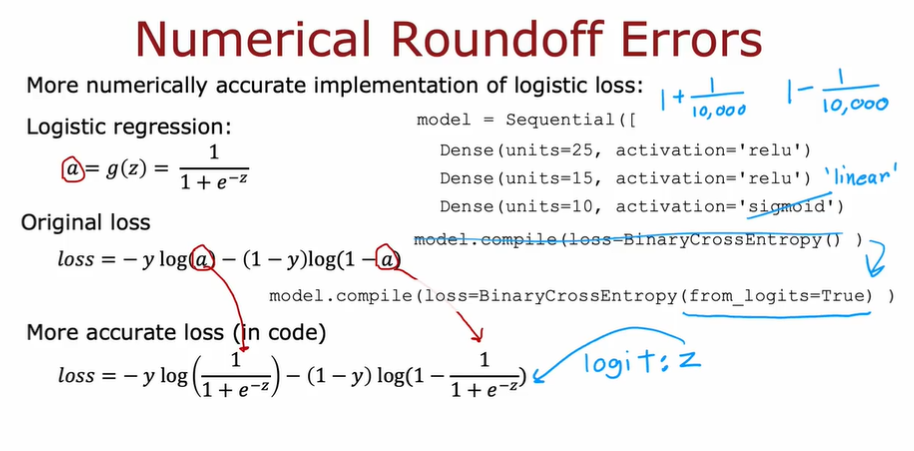• 在输出层使用 linear 激活函数（目的是为了不先计算 a/z，而是把整个式子放到最后再统一计算，避免了计算中间值而带来的精度误差），然后在 compile 选项里加上from_logits=True

### 3.8.2. 多标签分类问题

• multi-label classification problem
• 输出是一个标签向量，表示是否包含某个向量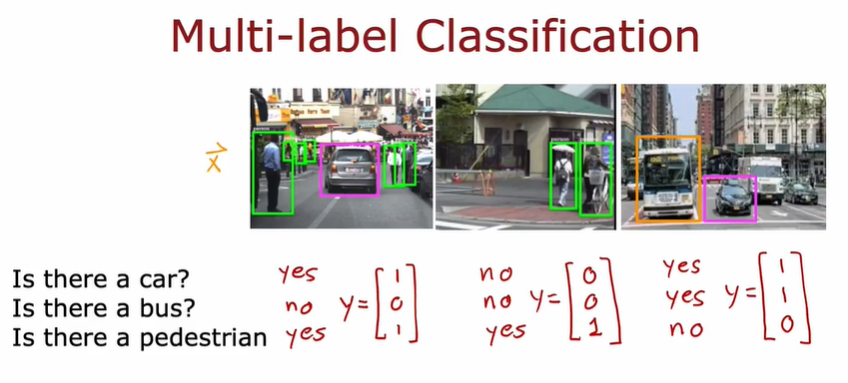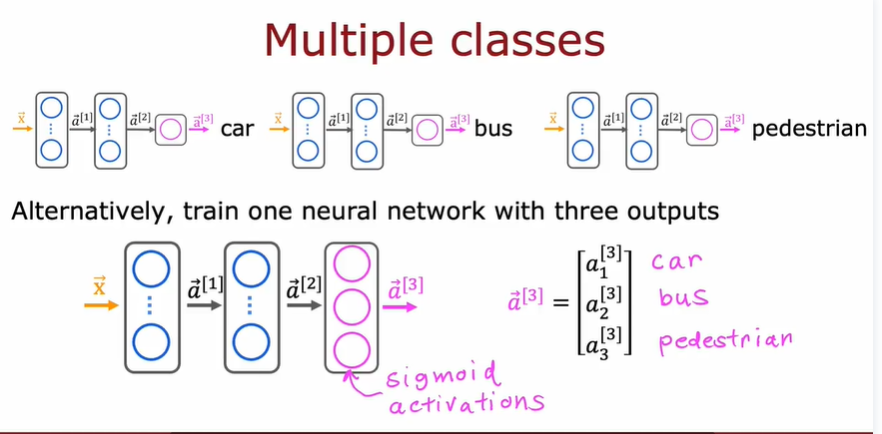• 可以自动调整学习率$\alpha$

• 对于不同模型使用不同$\alpha$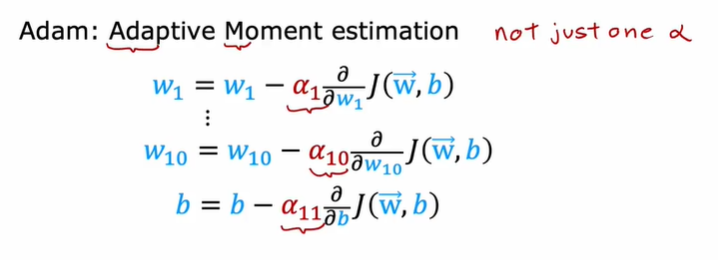## 3.10. Layer Types

### 3.10.2. Convolutional Layer

• 每一个神经元只关注**part of**前一层的输出

• 优点：

• 计算更快
• 所需的训练数据更少，也更不容易过拟合
• 例子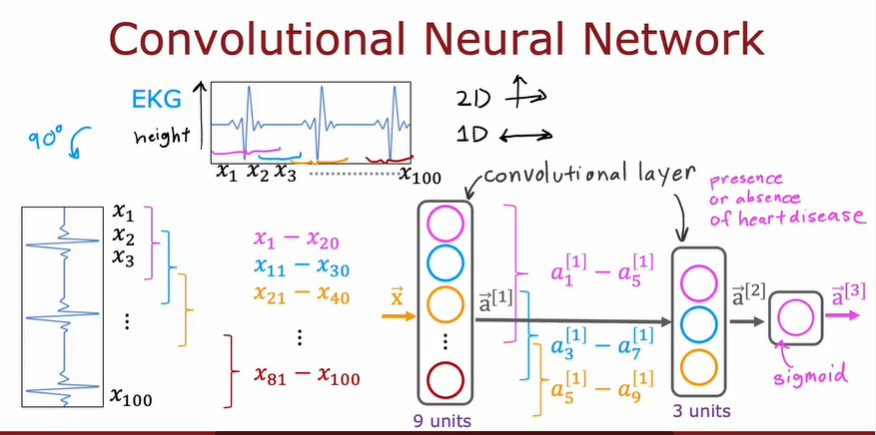• 可选择参数：每层的神经元数，每个神经元得到的输入数量